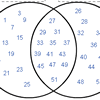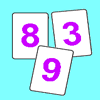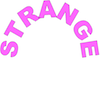Search by Topic

Resources tagged with Prime numbers similar to Various Venns:

Filter by: Content type:
Age range:
Challenge level:

There are 17 results

Broad Topics > Numbers and the Number System > Prime numbersVarious Venns

Age 7 to 11 Challenge Level:

Use the interactivities to complete these Venn diagrams.Code Breaker

Age 7 to 11 Challenge Level:

This problem is based on a code using two different prime numbers less than 10. You'll need to multiply them together and shift the alphabet forwards by the result. Can you decipher the code?Factors and Multiples Game for Two

Age 7 to 14 Challenge Level:

Factors and Multiples game for an adult and child. How can you make sure you win this game?Factors and Multiples Game

Age 7 to 16 Challenge Level:

A game in which players take it in turns to choose a number. Can you block your opponent?Stars

Age 11 to 14 Challenge Level:

Can you find a relationship between the number of dots on the circle and the number of steps that will ensure that all points are hit?Coins

Age 11 to 14 Challenge Level:

A man has 5 coins in his pocket. Given the clues, can you work out what the coins are?Factor Lines

Age 7 to 14 Challenge Level:

Arrange the four number cards on the grid, according to the rules, to make a diagonal, vertical or horizontal line.Prompt Cards

Age 7 to 11 Challenge Level:

These two group activities use mathematical reasoning - one is numerical, one geometric.Two Primes Make One Square

Age 7 to 11 Challenge Level:

Can you make square numbers by adding two prime numbers together?Factors and Multiples Puzzle

Age 11 to 14 Challenge Level:

Using your knowledge of the properties of numbers, can you fill all the squares on the board?Penta Primes

Age 7 to 11 Challenge Level:

Using all ten cards from 0 to 9, rearrange them to make five prime numbers. Can you find any other ways of doing it?Tom's Number

Age 7 to 11 Challenge Level:

Work out Tom's number from the answers he gives his friend. He will only answer 'yes' or 'no'.Round and Round the Circle

Age 7 to 11 Challenge Level:

What happens if you join every second point on this circle? How about every third point? Try with different steps and see if you can predict what will happen.Swimming Pool Tiles

Age 7 to 11 Challenge Level:

This activity creates an opportunity to explore all kinds of number-related patterns.A Conversation Piece

Age 7 to 11 Challenge Level:

Take the number 6 469 693 230 and divide it by the first ten prime numbers and you'll find the most beautiful, most magic of all numbers. What is it?Sieve of Eratosthenes

Age 11 to 14 Challenge Level:

Follow this recipe for sieving numbers and see what interesting patterns emerge.Strange Numbers

Age 11 to 14 Challenge Level:

All strange numbers are prime. Every one digit prime number is strange and a number of two or more digits is strange if and only if so are the two numbers obtained from it by omitting either. . . .